Next: Specific heats Up: Applications of statistical thermodynamics Previous: The equipartition theorem

## Harmonic oscillators

Our proof of the equipartition theorem depends crucially on the classical approximation. To see how quantum effects modify this result, let us examine a particularly simple system which we know how to analyze using both classical and quantum physics: i.e., a simple harmonic oscillator. Consider a one-dimensional harmonic oscillator in equilibrium with a heat reservoir at temperature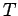. The energy of the oscillator is given by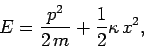(467)

where the first term on the right-hand side is the kinetic energy, involving the momentum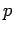and mass, and the second term is the potential energy, involving the displacementand the force constant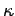. Each of these terms is quadratic in the respective variable. So, in the classical approximation the equipartition theorem yields: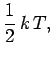(468)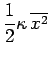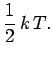(469)

That is, the mean kinetic energy of the oscillator is equal to the mean potential energy which equals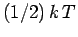. It follows that the mean total energy is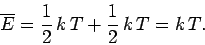(470)

According to quantum mechanics, the energy levels of a harmonic oscillator are equally spaced and satisfy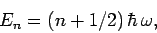(471)

whereis a non-negative integer, and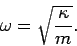(472)

The partition function for such an oscillator is given by(473)

Now,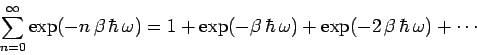(474)

is simply the sum of an infinite geometric series, and can be evaluated immediately,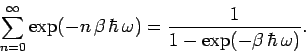(475)

Thus, the partition function takes the form(476)

and(477)

The mean energy of the oscillator is given by [see Eq. (399)]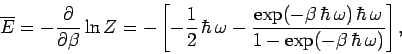(478)

or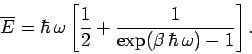(479)

Consider the limit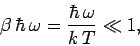(480)

in which the thermal energyis large compared to the separationbetween the energy levels. In this limit,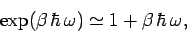(481)

so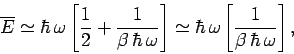(482)

giving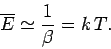(483)

Thus, the classical result (470) holds whenever the thermal energy greatly exceeds the typical spacing between quantum energy levels.

Consider the limit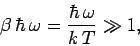(484)

in which the thermal energy is small compared to the separation between the energy levels. In this limit,(485)

and so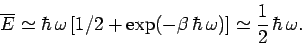(486)

Thus, if the thermal energy is much less than the spacing between quantum states then the mean energy approaches that of the ground-state (the so-called zero point energy). Clearly, the equipartition theorem is only valid in the former limit, where, and the oscillator possess sufficient thermal energy to explore many of its possible quantum states.Next: Specific heats Up: Applications of statistical thermodynamics Previous: The equipartition theorem
Richard Fitzpatrick 2006-02-02